# Math Matching Games

Students of all grades can play these fun and interactive math matching games to practice key mathematical concepts while improving their memory in the same time. The games are sorted by grade level.

### Concentration Games

In these games, students click on two cards to match a multiplication question with the correct answer. If there is a match, the problems remain on the page; if not, the cards are turned over. Click on the following links to play these fun games.One-Digit Addition Game
One-Digit Plus Two-Digit Numbers Addition Game

### Multiplication Concentration GamesTime Matching Game

This game is suitable for 2nd and 3rd grade students. For each question, kids will tell the time to the nearest 5 minutes. by matching the analog clocks with the correct digital clocks.Another Time Matching Game

This game is suitable for 3rd grade students and English language learners of all ages. The object of each problem is to match the analog clocks with the correct phrase.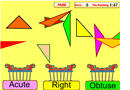Classifying Triangles Game

In this fun game, students will drag and drop different triangles in the correct bin in less than two minutes.Classifying Geometric Figures Game

In this fast-paced game, kids will have fun classifying plane and space geometrical figures.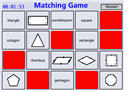2D Shapes Game (Concentration)

In this game students click on two cards to match the figure of a two-dimensional shape with its name. If there is a match, the problems remain on the page; if not, the cards are turned over.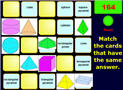3D Shapes Game (Concentration)

Have fun matching pictures of three-dimensional shapes with the correct words. If there is a match, the problems remain on the page; if not, the cards are turned over.Polygon or Not?

In this sorting game, students must classify different figures as polygons or not polygons. The game must be completed in 90 seconds.Changing Fractions to Percents

In this concentration game, students will match different fractions with the equivalent percents.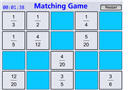Equivalent Fractions Game

In this concentration game, students click on two cards to match equivalent fractions. If there is a match, the problems remain on the page; if not, the cards are turned over.Adding Integers Game

Do you know how to add integers? Play this interactive game to classify different sums as positive, negative, or zero.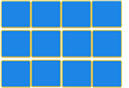Adding Integers Concentration Game

Have fun solving addition problems with integers.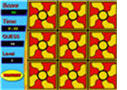Square Root Game

In this interactive concentration game, students will match the square roots of perfect squares with the correct numbers.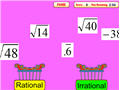Rational and Irrational Numbers

In this interesting game, middle school students will have fun classifying rational and irrational numbers.Set Theory Matching Game

In this concentration game, students will match mathematical symbols used in set theory with the corresponding vocabulary words.

Return from the Math Matching Games page to the Math Play homepage.